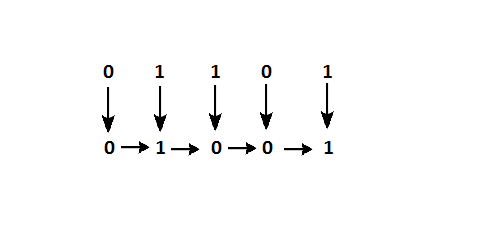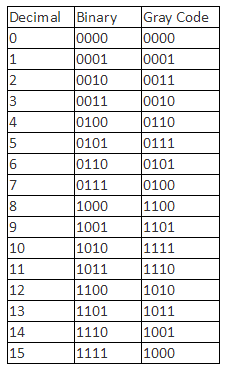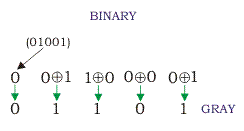# What is Grey Code? Binary to Grey and Grey to Binary Conversions

Gray Code is one of the most important codes. It is a non-weighted code which belongs to a class of codes called minimum change codes. In this codes while traversing from one step to another step only one bit in the code group changes. In case of Gray Code two adjacent code numbers differs from each other by only one bit. The idea of it can be cleared from the table given below.  As in the case of this code any types of arithmetical operations is not applicable but it has some applications in analog to digital converters and in some input/output devices.

GRAY CODES are non weighted codes , that is they can’t be provided a weight to calculate their equivalent in decimal . Gray codes are often called reflected binary code, the reason is clear if you compare the column of gray code with the binary code . gray Code is a symbolic representation of discrete information. Codes are of different types. Gray Code is one of the most important codes. It is a non-weighted code which belongs to a class of codes called minimum change codes. In this codes while traversing from one step to another step only one bit in the code group changes. In case of Gray Code two adjacent code numbers differs from each other by only one bit. The idea of it can be cleared from the table given below sections.

## Gray to Binary Code Converter

Gray code to binary conversion is very simple and easy process. Following steps can make your idea clear on this type of conversions.

1. The M.S.B of the binary number will be equal to the M.S.B of the given gray code.
2. Now if the second gray bit is 0 the second binary bit will be same as the previous or the first bit. If the gray bit is 1 the second binary bit will alter. If it was 1 it will be 0 and if it was 0 it will be 1.
3. This step is continued for all the bits to do Gray code to binary conversion. Let the gray code be 01101The M.S.B of the binary will be 0 as the M.S.B of gray is 0. Now move to the next gray bit. As it is 1 the previous binary bit will alter i.e it will be 1, thus the second binary bit will be 1. Next look at the third bit of the gray code. It is again 1 thus the previous bit i.e the second binary bit will again alter and the third bit of the binary number will be 0. Now, 4th bit of the given gray is 0 so the previous binary bit will be unchanged, i.e 4th binary bit will be 0. Now again the 5th grey bit is 1 thus the previous binary bit will alter, it will be 1 from 0. Therefore the equivalent Binary number in case of gray code to binary conversion will be (01001)## Binary to gray code conversion

As you can see from below example that Binary to gray code conversion is a very simple process. There are several steps to do this types of conversions. Steps given below elaborate on the idea on this type of conversion.

1. The M.S.B. of the gray code will be exactly equal to the first bit of the given binary number.
2. Now the second bit of the code will be exclusive-or of the first and second bit of the given binary number, i.e if both the bits are same the result will be 0 and if they are different the result will be 1.
3. The third bit of gray code will be equal to the exclusive-or of the second and third bit of the given binary number. Thus the Binary to gray code conversion goes on. One example given below can make your idea clear on this type of conversion.So it is resulted that the equivalent gray code for binary number 01001 is 01101. Now concentrate on the example where the M.S.B. of the binary is 0 so for it will be 0 for the most significant gray bit. Next, the XOR of the first and the second bit is done. The bits are different so the resultant gray bit will be 1. Again move to the next step, XOR of second and third bit is again 1 as they are different. Next, XOR of third and fourth bit is 0 as both the bits are same. Lastly the XOR of fourth and fifth bit is 1 as they are different. That is how the result of binary to gray code conversion of 01001 is done whose equivalent gray code is 01101.

error: Content is protected !!